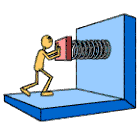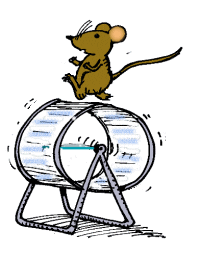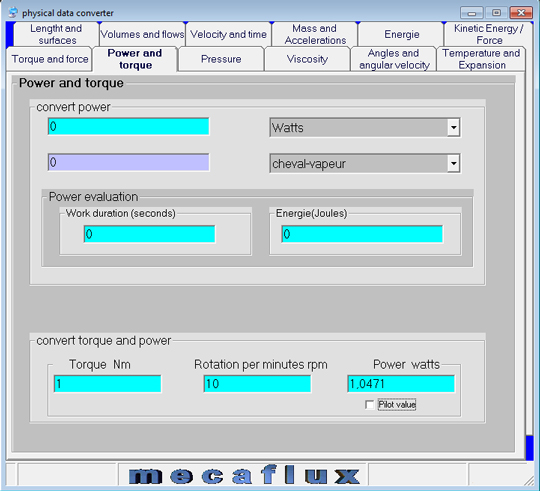The power calculation,power of a wind turbine, engine power, electrical power, or mechanical power, links an amount of energy (work) to a duration (time) .

The international unit of power is the watt, the multiple is the kilowatt

1 watt (power) = 1 joule (work) applied for one second (duration)

the unit of work is the Joule

the work is the result of a force applied to an object multiplied by the distance traveled by the object, during application of the force. We consider that this force is applied in the direction of the movement.Work (joule)=Force (newton) x Distance (meter)

If the direction of this force forms an angle with the direction of motion we will take

Work (joule) = Force (newton) x Distance (meter) x cosinus angle

Work in joules, distance in meters, Force in Newtons

• The power of an engine running is given by the torque and rotational speed. Power (W) = Torque (Nm) * rotation speed (Rad/s)

If the mouse exerts 1 Nm of torque to turn the cage to 1 radian / second, it provides a power of 1 watt.Reminder: 1 rad/sec / (2 * pi) = 1 rev/sec

For conversions of various forms of power we use the integrated converter to mecaflux Standard: